# How to Type Minus Sign in Excel Without Formula (6 Simple Methods)

Get FREE Advanced Excel Exercises with Solutions!

This tutorial will illustrate simple methods of how to type minus sign in Excel without a formula. Suppose we are trying to type a minus sign in a cell. In that case, Excel considers it as a formula. So, we get an error in return. To solve this problem we will see how we can type minus sign in Excel. But, we will do it without using any formula.

## 6 Simple Methods to Type Minus Sign in Excel Without Formula

Throughout this article, we will show 6 simple methods to type minus sign in Excel without a formula. To explain these methods we will use the following dataset. In the dataset, we have 5 numbers. Using different methods we will type minus signs before them. In some examples, we will create a new column containing the numbers with a minus sign. But, in other examples, we will overwrite the existing values with minus signs.### 1. Apply Insert Tab to Type Minus Sign in Excel Without Formula

First and foremost, we will use the Insert tab to type minus sign in Excel without a formula. In the following dataset, we’ll insert the minus sign to type the number of cells (B5:B8) into cells (C5:C8).Let’s see the steps to perform this method.

STEPS:

• To begin with, select cell C5.• In addition, go to the Insert tab. From the Symbols section of the ribbon, select Symbol.• A new dialogue box named Symbol will appear.
• Furthermore, select the ‘Mathematical Operators’ in the subset section.
• Then, select the symbol Minus Sign.• The above command will input a minus in cell C5.• After that, type 7 in cell C5.• Finally, insert minus sign in the same way for the other values.### 2. Insert Minus Sign in Excel Without Formula Using Single Quote

In the second method, we will insert minus sign in Excel using a single quote before any number. To do this follow the below steps.

STEPS:

• First, type 7 in cell C5.
• Add a single quote before it.• Press Enter.
• We can see an error in cell C5.
• Next, click on the small icon left.
• Then, select the option ‘Convert to Number’.• The above command returns the value of cell B5 with a minus sign.• After that, repeat the above steps for all the values.
• So, we get results like the following image.### 3. Add Custom Formatting to Type Minus Sign Without Excel Formula

Adding custom formatting is very convenient if we want to type minus sign in Excel without a formula. In the following dataset, we will insert minus sign before the existing numeric values.Let’s see the steps to perform this method.

STEPS:

• Firstly, select cell (B5:B8).
• Secondly, right-click on the selected region. Select the option ‘Format Cells’.• A new dialogue box named Format Cells will appear.
• Thirdly, in the dialogue box go to the Number
• Select the option Custom from the category section.
• Next, in the Type input field insert -#,###, #,###. This will give us a preview of the output in the Sample.
• Then, click on OK.• Lastly, we can see our desired result in the following image.

### 4. Excel Paste Special Multiplication for Typing Minus Sign

In this method, we’ll use the value multiplication from the Paste Special options to type minus sign in Excel. In the following dataset, we can see the value of the multiplier is -1.Follow the below steps to perform this action.

STEPS:

• In the beginning, select cell D5.
• Next, press Ctrl + C to copy that value.• Afterward, select cells (B5:B8).
• Then, select the option Paste Special.• A new dialogue box will appear like the following image.
• After that, check the option Multiply from the Operation section.
• Now, click on OK.• As a result, we get minus signs before all the values.### 5. Type Minus Sign in Excel Without Formula Using Flash Fill Feature

Flash Fill can detect a data pattern and fill cells with data following that pattern. In this method, we will use Flash Fill to type minus sign in excel without a formula. To do this we will follow the below steps.

STEPS:

• To begin with, type the desired value of cell B5 in cell C5 with a minus sign.

###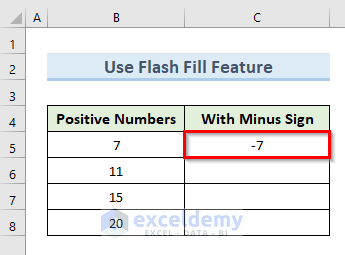• In addition, select cell C6.
• Press Ctrl + E.
• The above action fills cells (C6:C10).
• Furthermore, click the small icon left.
• Then, select the option ‘Accept suggestions’.

###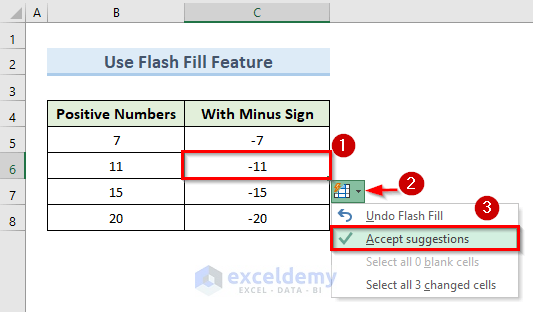• In the end, we get numeric values with minus signs before them.

### 6. Apply VBA Code to Type Minus Sign in Excel Without Formula

If you are an advanced excel user, you can use VBA to type minus sign in excel. Using VBA has been always quick and easy to accomplish a task in Excel. To apply VBA in this method we will go with the following steps.

STEPS:

• Firstly, select cells (B5:B8).

##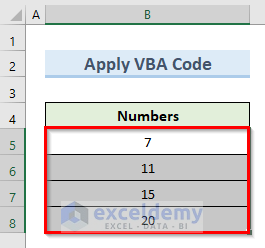• Secondly, go to the Developer tab.
• Then, select the option Visual Macros from the ribbon.

##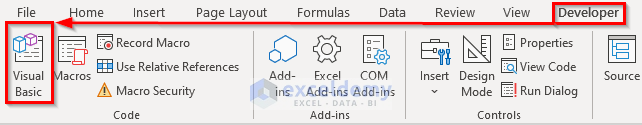• A new VBA project window will open.
• Thirdly, in that window, right-click on the active sheet named ‘Sheet7 (VBA)’.
• Then, select Insert > Module.

##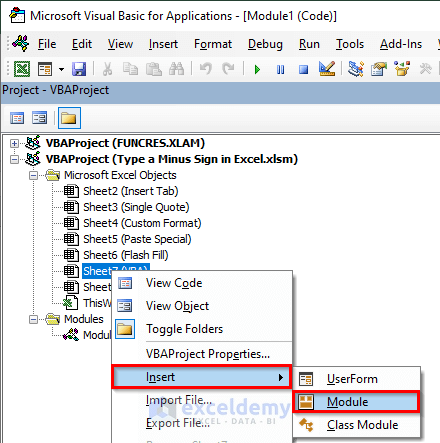• A new VBA code window will open.
• Insert the following code in that code window:
``````Sub TypeMinusSign()
For Each Cell In Selection
If Cell.Value > 0 Then
Cell.Value = -Cell.Value
End If
Next Cell
End Sub``````
• Now, click on OK or press the F5 key to run the code.

##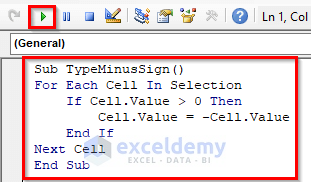• Lastly, we can see minus signs before the selected numbers in our dataset.

## Related ArticlesMukesh Dipto

Hi there! I am Mukesh Dipto. I graduated with a bachelor's degree in engineering. Currently, I am working as a technical content writer in ExcelDemy. You will find all my articles on Microsoft Excel on this site. Outside of the workplace, my hobbies and interests include sports and meeting new people. I also enjoy sports. My favorite sports are football (to watch and play) and Badminton (play).

We will be happy to hear your thoughtsAdvanced Excel Exercises with Solutions PDF